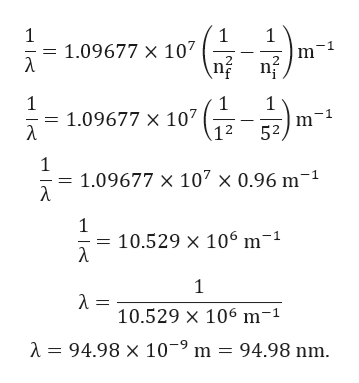# How do you calculate the wavelength of the light emitted by a hydrogen atom during a transition of its electron from the n = 5 to the n = 1 principal energy level

Question
24 views

How do you calculate the wavelength of the light emitted by a hydrogen atom during a transition of its electron from the n = 5 to the n = 1 principal energy level

check_circle

Step 1

Given:

Higher energy state (ni) = 5.

Lower energy state (nf) = 1.

Step 2

Rydberg equation is given by:

Step 3

Calculation for w...help_outlineImage Transcriptionclose1 1 1.09677 x 107 \nf 1 1 1.09677 X 107 1 m 1 12 52, 1 1 1.09677 x 107 x 0.96 m 1 1 = 10.529 x 106 m1 1 λ- 10.529 x 106 m-1 94.98 x 109 m = 94.98 nm. fullscreen

### Want to see the full answer?

See Solution

#### Want to see this answer and more?

Solutions are written by subject experts who are available 24/7. Questions are typically answered within 1 hour.*

See Solution
*Response times may vary by subject and question.
Tagged in

### Atomic structure